### IMO Shortlist 2012 problem G1

Kvaliteta:
Avg: 0,0
Težina:
Avg: 5,7
Given triangle$ABC$ the point$J$ is the centre of the excircle opposite the vertex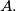$A.$ This excircle is tangent to the side$BC$ at$M$, and to the lines$AB$ and$AC$ at$K$ and$L$, respectively. The lines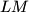$LM$ and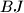$BJ$ meet at$F$, and the lines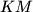$KM$ and$CJ$ meet at$G.$ Let$S$ be the point of intersection of the lines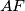$AF$ and$BC$, and let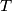$T$ be the point of intersection of the lines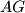$AG$ and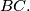$BC.$ Prove that$M$ is the midpoint of$ST.$

(The excircle of$ABC$ opposite the vertex$A$ is the circle that is tangent to the line segment$BC$, to the ray$AB$ beyond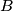$B$, and to the ray$AC$ beyond$C$.)

Proposed by Evangelos Psychas, Greece
Izvor: Međunarodna matematička olimpijada, shortlist 2012